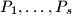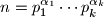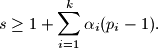IMO Shortlist 2010 problem C7

Kvaliteta:
Avg: 0,0
Težina:
Avg: 9,0
Let$P_1, \ldots , P_s$ be arithmetic progressions of integers, the following conditions being satisfied:

(i) each integer belongs to at least one of them;
(ii) each progression contains a number which does not belong to other progressions.

Denote by$n$ the least common multiple of the ratios of these progressions; let$n=p_1^{\alpha_1} \cdots p_k^{\alpha_k}$ its prime factorization.

Prove thatProposed by Dierk Schleicher, Germany
Izvor: Međunarodna matematička olimpijada, shortlist 2010